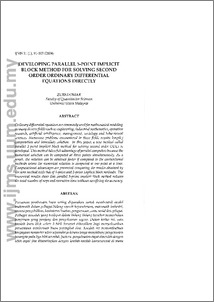mailto:uumlib@uum.edu.my 24x7 Service; AnyTime; AnyWhere

# Developing parallel 3-point implicit block method for solving second order ordinary differential equations directly

Omar, Zurni (2004) Developing parallel 3-point implicit block method for solving second order ordinary differential equations directly. International Journal of Management Studies (IJMS), 11 (1). pp. 91-103. ISSN 2180-2467Preview PDF Download (17MB) | Preview
Official URL: http://ijms.uum.edu.my

## Abstract

Ordinary differential equations are commonly used for mathematical modeling in many diverse fields such as engineering, industrial mathematics, operation research, artificial intelligence, management, sociology and behavioural sciences. Numerous problems encountered in these fields require lengthy computation and immediate solution. In this paper, a new method called parallel 3-point implicit block method for solving second order ODES is developed. This method takes full advantage of parallel computers because the numerical solution can be computed at three points simultaneously. As a result, the solution can be obtained faster if compared to the conventional methods where the numerical solution is computed at one point at a time. Computational advantages are presented comparing the results obtained by the new method with that of 1-point and 2-point implicit block methods. The numerical results show that parallel 3-point implicit block method reduces the total number of steps and execution time without sacrificing the accuracy.

Item Type: Article Differential equations Q Science > QA Mathematics College of Arts and Sciences Prof. Dr. Zurni Omar 16 Jun 2010 16:26 16 Jun 2010 16:26 https://repo.uum.edu.my/id/eprint/11View Item